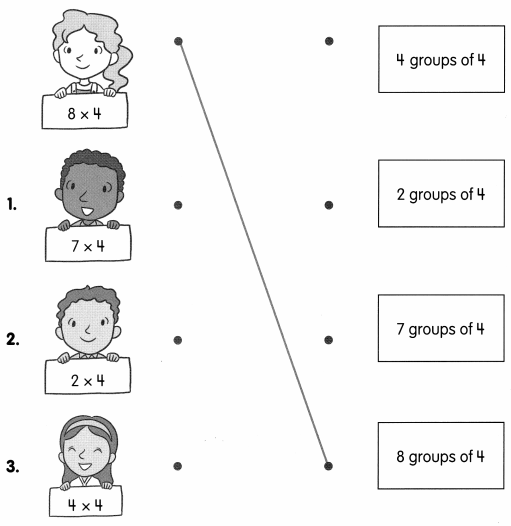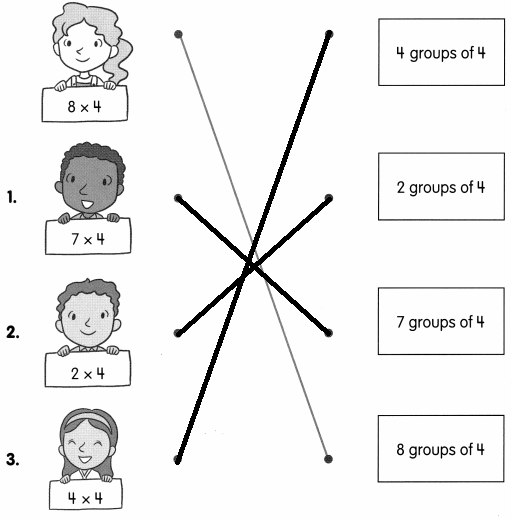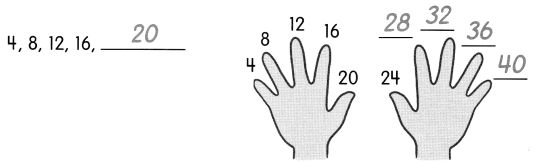# Math in Focus Grade 2 Chapter 15 Practice 3 Answer Key Multiplying 4: Skip-Counting

This handy Math in Focus Grade 2 Workbook Answer Key Chapter 15 Practice 3 Multiplying 4: Skip-Counting detailed solutions for the textbook questions.

## Math in Focus Grade 2 Chapter 15 Practice 3 Answer Key Multiplying 4: Skip-Counting

Match.Count by 4s. Then fill in the blanks.

ExampleQuestion 4.
12, 16, 20, __________, __________, __________, __________
12,16,20,24,28,32,36

Question 5.
16, __________, 24, 28, __________, __________, 40
16,20,24,28,32,36,40

Fill in the blanks.

Question 6.
3 × 4 = __________
3 x 4 = 12

Question 7.
6 × 4 = __________
6 x 4 = 24

Question 8.
2 × 4 = __________
2 x 4 = 8

Question 9.
8 × 4 = __________
8 x 4 = 32

Question 10.
9 × 4 = __________
9 x 4 = 36

Question 11.
4 × 4 = __________
4 x 4 = 16

Question 12.
10 × 4 = __________
10 x 4 = 40

Question 13.
7 × 4 = __________